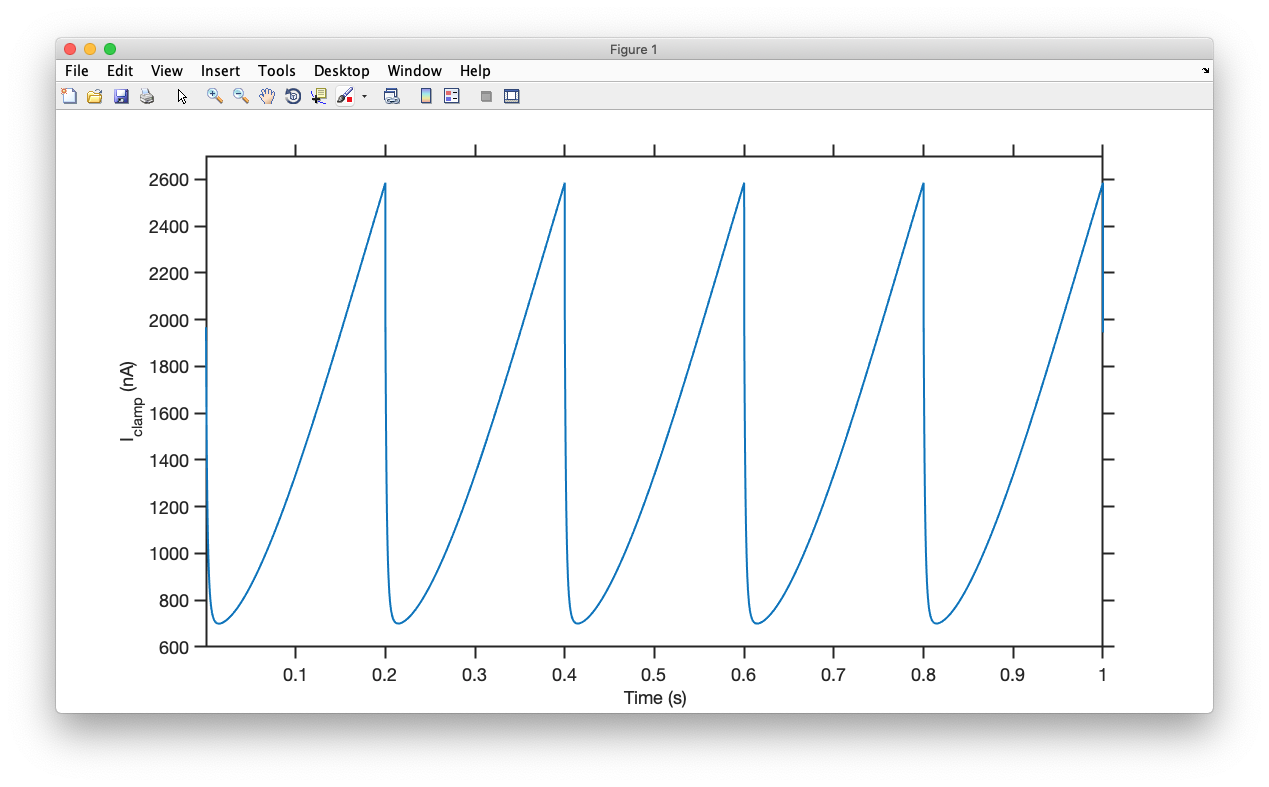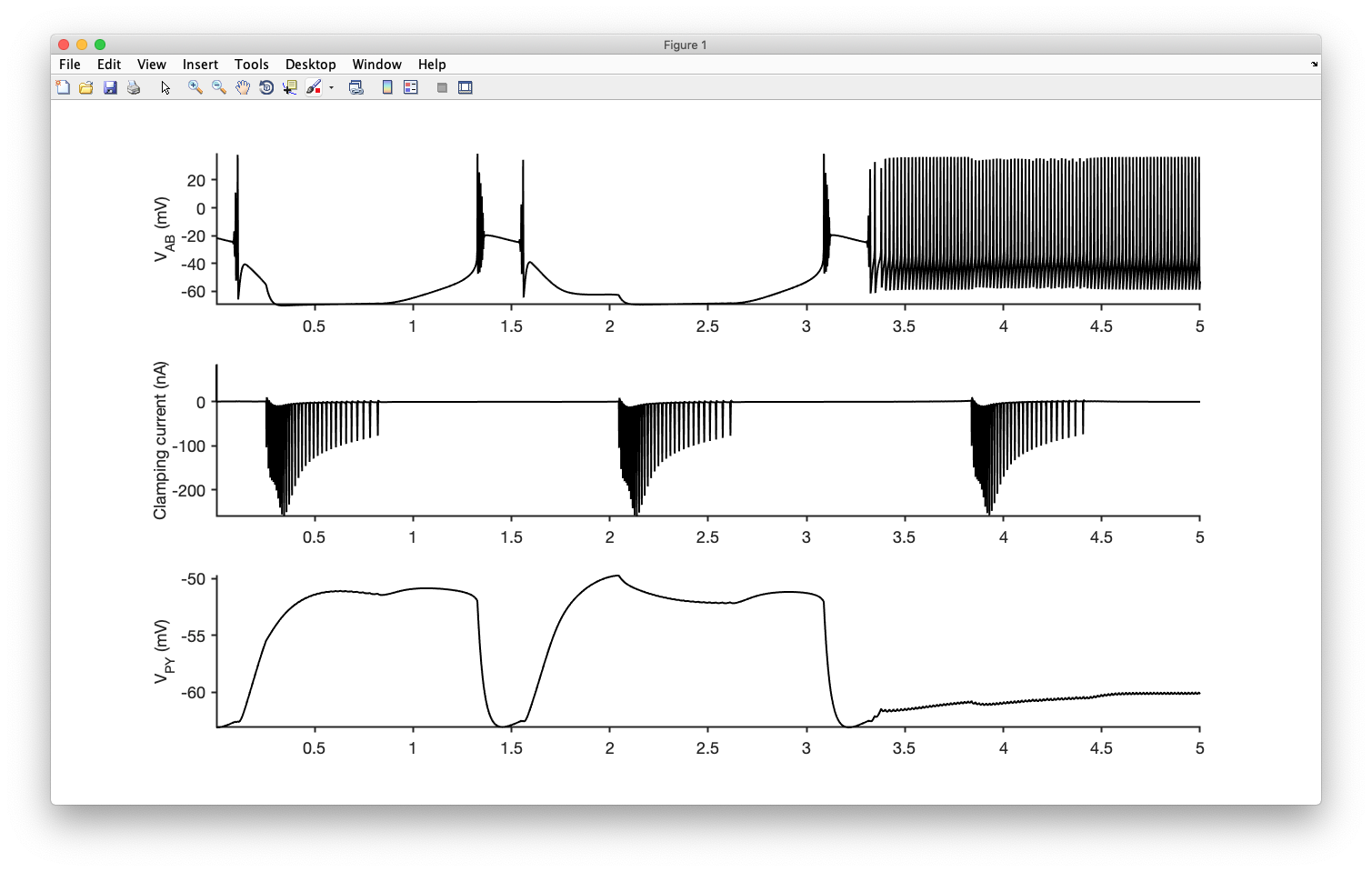# Voltage clamp a compartment

This document describes how to voltage clamp a compartment in xolotl.

## Introduction and Limitations¶

Voltage clamping is a technique where you insert an electrode into a cell (or part of a cell), and use a computer to inject whatever current is needed to keep the voltage of that compartment at a desired value. Experimentally, the voltage can be monitored either using a second electrode or by rapidly switching the first electrode between modes where it is used to record the voltage or to inject current.

xolotl allows you to voltage clamp compartments with the following limitations:

1. You cannot simultaneously inject current and voltage clamp
2. Voltage clamping a single compartment in a multi-compartment model is not recommended, since xolotl does not use implicit solvers when voltage clamped.

## Voltage clamping a single compartment to a fixed value¶

Here, we will use a single-compartment model of a bursting neuron. You can create this model using:

x = xolotl.examples.neurons.BurstingNeuron;


Now, if we inspect the V_clamp property using x.V_clamp, we see:

x.V_clamp

ans =
NaN


that it is a NaN value. This means that the simulation will not run in voltage clamp mode. To voltage clamp the cell to -20mV, use:

x.V_clamp = -20;


Now, we can integrate the model to obtain the clamping current and plot that:

I = x.integrate;
time = (1:length(I))*x.dt*1e-3;
plot(time,I)
set(gca,'XScale','log')
ylabel('I_{clamp} (nA)')
xlabel('Time (s)')


We see something like this:## Clamping a cell to a time-varying signal¶

We can also clamp a cell to a time-varying signal, for example, a sawtooth wave:

V_clamp = rem(linspace(0,100,length(I)),20)-10;
x.V_clamp = V_clamp(:);


Integrating and plotting this yields a different current required to clamp the cell:## Voltage clamping a cell in a network¶

We can also voltage clamp a single cell in a network. To demonstrate this, we will use a 3-cell network of the pyloric circuit:

x = xolotl.examples.networks.pyloric;


Here, let's voltage clamp one cell to another cell's voltage dynamics.

V = x.integrate;
x.V_clamp  = V*NaN;
x.V_clamp(:,2) = V(:,1);


Now, when we integrate it using:

data = x.integrate;


Warning

Note that the voltage and the clamping current are returned packaged into the same variable!

Let's tease them apart and plot them:

figure; hold on
time = (1:length(data))*x.dt*1e-3;
subplot(3,1,1); hold on
ylabel('V_{AB} (mV)')
plot(time,data(:,1),'k')
subplot(3,1,2); hold on
plot(time,data(:,2),'k')
ylabel('Clamping current (nA)')
subplot(3,1,3); hold on
plot(time,data(:,3),'k')
ylabel('V_{PY} (mV)')


We see something like this:## Voltage clamping multiple compartments¶

To voltage clamp more than one compartment, fill out more than one column of the x.V_clamp property with non-NaN values.

## Voltage clamp and integration¶

When voltage clamp is used with single-compartment or network models (no electrical synapses), either the default exponential Euler or Runge-Kutta integration methods can be used. Both are compatible with voltage clamp and yield nearly identical results.

As usual, you can switch between integration methods by setting the solver order property.

Be careful when using voltage clamp on multi-compartment models, since it is not possible to use implicit solvers when doing so.